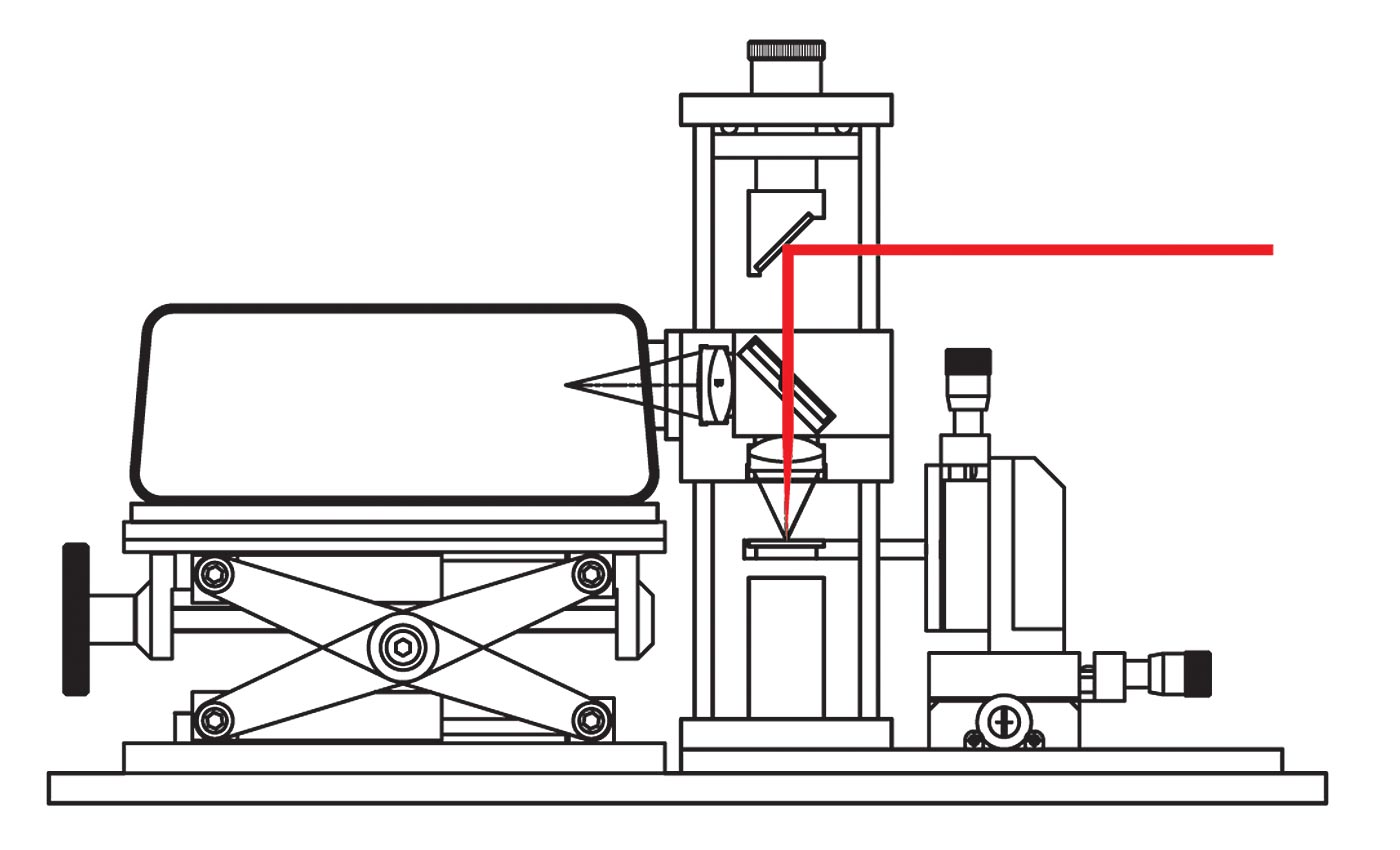Second Harmonic Generation

Model : HO-ED-LOE-05

The objective of this experiment is to observe non-linear effect, second harmonic generation in 2nd order non linear crystals. Second harmonic generation (also called frequency doubling or abbreviated SHG) is a nonlinear optical process, in which photons with the same frequency interacting with a nonlinear material are effectively "combined" to generate new photons with twice the energy, and therefore twice the frequency and half the wavelength of the initial photons.

Due to nonlinear atomic polarization, crystals can emit a different frequency of light other than absorbed. In order to see the second order effect of atomic polarization, which causes doubling in frequency of the incident light, the incident light must be intense and coherent source, like a laser. Potassium Dihydrogen Phosphate (KDP), Urea etc. are the crystals that exhibit nonlinear polarization properties. When light hits a crystal, it excites the atomic dipoles. In classical optics, it is assumed that this polarization of atoms is linear to the magnitude of electrical field of the incident light. In reality, this polarization is a nonlinear phenomenon, but the nonlinear components are evident only for high intensity.

Experiment Examples

Potassium Dihydrogen Phosphate (KDP) crystal growth

The two measurable quantities connected with the z - scan are non linear absorption and non linear refraction. These parameters are associated with the imaginary and real part of the third order non linear susceptibility and provide important information about the properties of the material.

Second harmonic generation of light - frequency doubling in Urea and KDP crystals

The 'Beam Diameter' is defined as "The distance across the center of the beam for which the irradiance (E) equals (  1  /  e²  =  0.135  )". The 'Spot Size' (ω ) of the beam is "The radial distance (radius) from the center point of maximum irradiance to the 1  /  e² point". These definitions provide standard measures of laser beam size.

Comparison of SHG efficiency of urea and KDP crystals using a CCD spectrometer

The 'Beam Diameter' is defined as "The distance across the center of the beam for which the irradiance (E) equals (  1  /  e²  =  0.135  )". The 'Spot Size' (ω ) of the beam is "The radial distance (radius) from the center point of maximum irradiance to the 1  /  e² point". These definitions provide standard measures of laser beam size.Fig. Experiment setup

In order for the second harmonic light to be visible in the output beam from the crystal, the atomic dipoles must be radiating constructively. This means that the second harmonic light output must be in phase with the incident laser light. The phase of the field at ω needs to be matched with the phase at 2ω in second harmonic generation. This is critical so that they do not interfere destructively. The applied electric field is strong enough such that it produces the second order radiation but the first order radiation does not become the dominant one. Hence, it can be thought of as a superposition of signals at two frequencies which should be added and not cancelled out.

In this experiment, a non centro symmetric crystal is probed with infrared laser to produce visible light. This experiment shows that frequency doubling can be achieved in urea and lab grown KDP crystals. Using a high power infrared laser we can see frequency doubling in crystals. Efficiency of emission from each crystal is calculated using standard techniques.

Feel free to contact our engineers for any assistance|